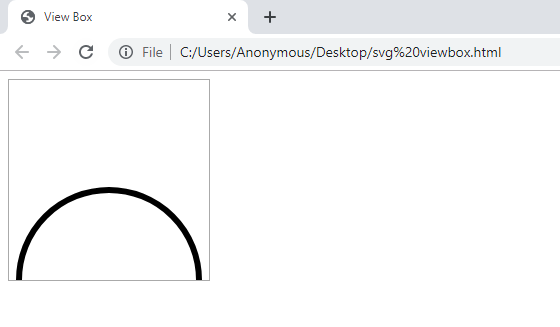Open In App

# SVG viewBox Attribute

The viewBox is an attribute of the SVG element in HTML. It is used to scale the SVG element that means we can set the coordinates as well as width and height.

Syntax:

`viewBox = "min-x min-y width height"`

Attribute Values:

• min-x: It is used to set the horizontal axis. It is used to make the SVG move on a horizontal axis (i.e Left and Right).
• min-y: It is used to set the vertical axis. It is used to make the SVG move on a vertical axis (i.e Up and Down).
• width: It is used to set the width of viewbox.
• height: It is used to set the height of viewbox.

Note: The letter ‘B’ is capital in the viewBox. So with the help of these values, we can scale the SVG vector and change the direction of it (i.e make it to left, right, top or bottom) based on the value defined in the width and height property in the SVG element.

Example:

## html

 ```<``html``>` `<``head``>``    ``<``title``>SVG viewBox Attribute``    ` `    ``<``style` `type="text/css">``        ``svg {``            ``border: 1px solid #aaa;``        ``}``    ````` `<``body``>``    ` `    ````    ``<``svg` `width="200" height="200">``        ``<``circle` `cx="50" cy="50" r="45"``            ``stroke="#000" stroke-width="3"``            ``fill="none"/>``    ````    ` `    ````    ``<``svg` `width="200" height="200" viewBox="0 0 200 200">``        ``<``circle` `cx="50" cy="50" r="45" stroke="#000"``            ``stroke-width="3" fill="none"/>``    ````` ``

Output: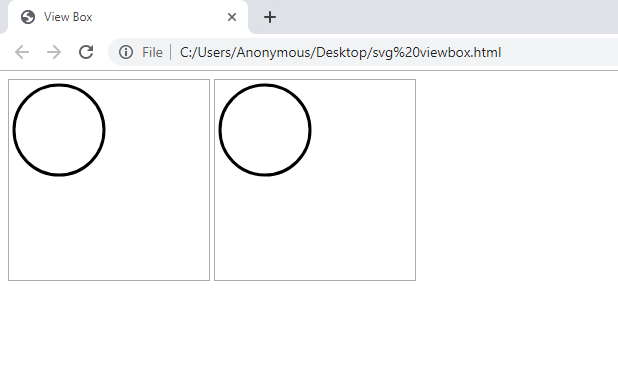Here, the square box shows the border for the SVG and with viewBox attribute we can set the scale and pan for the vector. The output for both of the above SVG elements are same. We set the width and height for SVG and viewBox equal (i.e 200) so we are getting both the circles of the same size.

Values of width and height: With the width and height values you can change the size of the SVG vector. So, if we want to change the size and make it larger, then set the value for width and height, in viewBox, smaller than the width and height properties of the SVG element.

Example:

## html

 ```<``html``>` `<``head``>``    ``<``title``>SVG viewBox Attribute``    ` `    ``<``style` `type="text/css">``        ``svg {``            ``border: 1px solid #aaa;``        ``}``    ````` `<``body``>` `    ``<``svg` `width="200" height="200" viewBox="0 0 100 100">` `    ``<``circle` `cx="50" cy="50" r="45" stroke="#000"``            ``stroke-width="3" fill="none"/>``    ````` ``

Output: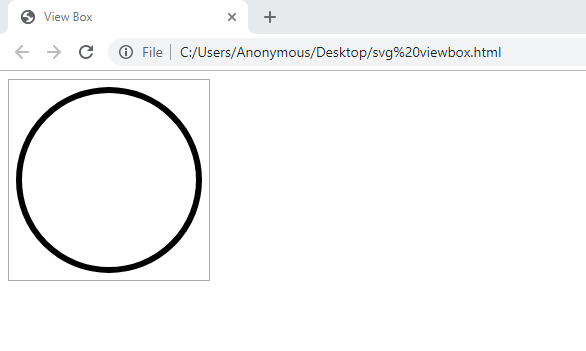So now, to change the size of the SVG vector and make it smaller we have to set the value of width and height in the viewBox, larger than the width and height properties of SVG element.

Example:

## html

 ```<``html``>` `<``head``>``    ``<``title``>SVG viewBox Attribute``    ` `    ``<``style` `type="text/css">``        ``svg {``            ``border: 1px solid #aaa;``        ``}``    ````` `<``body``>``    ``<``svg` `width="200" height="200" viewBox="0 0 300 300">` `    ``<``circle` `cx="50" cy="50" r="45" stroke="#000"``            ``stroke-width="3" fill="none"/>``    ````` ``

Output: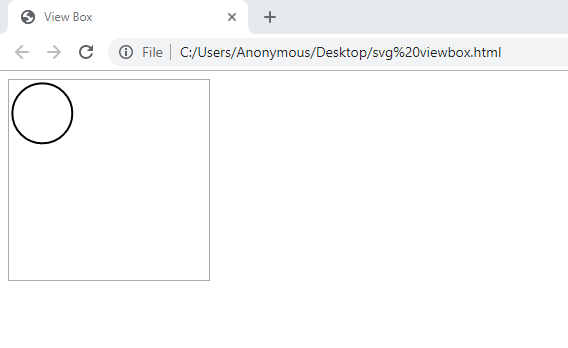Left Move: Set the value of x-min with a positive number. It will move the SVG in the left side.

Example:

## html

 ```<``html``>` `<``head``>``    ``<``title``>SVG viewBox Attribute``    ` `    ``<``style` `type="text/css">``        ``svg {``            ``border: 1px solid #aaa;``        ``}``    ````` `<``body``>``    ``<``svg` `width="200" height="200" viewBox="50 0 100 100">` `    ``<``circle` `cx="50" cy="50" r="45" stroke="#000"``            ``stroke-width="3" fill="none"/>``    ````` ``

Output: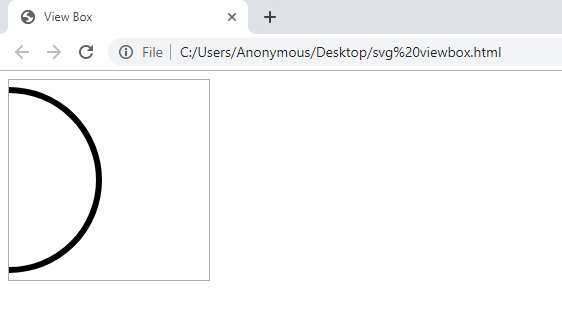Right Move: Set the value of x-min with a negative number. It will move the SVG to the right side.

Example:

## html

 ```<``html``>` `<``head``>``    ``<``title``>SVG viewBox Attribute``    ` `    ``<``style` `type="text/css">``        ``svg {``            ``border: 1px solid #aaa;``        ``}``    ````` `<``body``>``    ``<``svg` `width="200" height="200" viewBox="-50 0 100 100">` `    ``<``circle` `cx="50" cy="50" r="45" stroke="#000"``            ``stroke-width="3" fill="none"/>``    ````` ``

Output: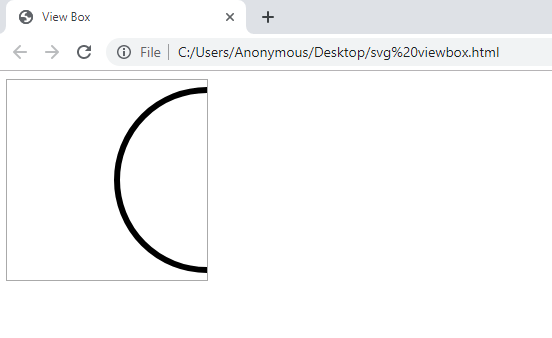Top Move: Set the value of y-min to a positive number. It will move the SVG on the top side.

Example:

## html

 ```<``html``>` `<``head``>``    ``<``title``>SVG viewBox Attribute``    ` `    ``<``style` `type="text/css">``        ``svg {``            ``border: 1px solid #aaa;``        ``}``    ````` `<``body``>``    ``<``svg` `width="200" height="200" viewBox="0 50 100 100">` `    ``<``circle` `cx="50" cy="50" r="45" stroke="#000"``            ``stroke-width="3" fill="none"/>``    ````` ``

Output: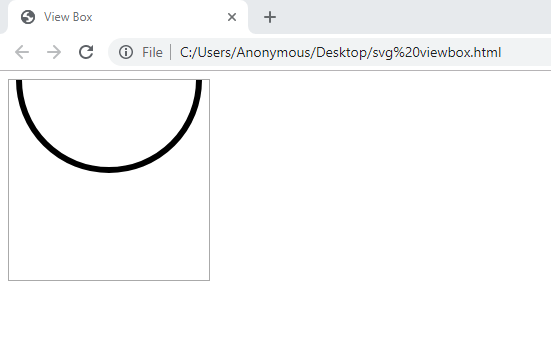Bottom Move: Set the value of y-min with a negative number. It will move the SVG on the bottom side.

Example:

## html

 ```<``html``>` `<``head``>``    ``<``title``>SVG viewBox Attribute``    ` `    ``<``style` `type="text/css">``        ``svg {``            ``border: 1px solid #aaa;``        ``}``    ````` `<``body``>``    ``<``svg` `width="200" height="200" viewBox="0 -50 100 100">` `    ``<``circle` `cx="50" cy="50" r="45" stroke="#000"``            ``stroke-width="3" fill="none"/>``    ````` ``

Output: